# Quiz 23: Price-Searcher Markets With Low Entry Barriers

Concept of price searchers market: Two common form of competitive market are - 1. Price takers market and 2. Price searchers market. Price takers market is an extreme form of competitive market. Here competition is so fierce that no firm can influence the price. They have to sell their product at market determined price. Another form of competitive market is known as price searchers market. In this market following properties are observed- a. There are many firm b. Products are little differentiated but close substitutes c. Entry barrier is low. So any new firm can enter easily. Demand curve of price searcher firm: Due to above properties a firm in price searchers market will face a downward sloping demand curve. If firm wants to sell more, then it has to reduce the selling price of last unit. Otherwise customer will not buy the product. Control on price fixation: Consider the property of close substitutes. Firms are manufacturing products that are almost same. But some differentiation is introducing by changing designs, packaging, location etc. Due to this close substitutability if a firm charges high price, then some customer will switch over to other firm. But some loyal customer will still buy the product at higher price. Thus firm has some control over the price. It can determine at what price the firm will sell its product. Cannot fix both price and quantity: However it does not mean that firm can settle both price and quantity at the same time. If firm has decided the price, then market forces will determine the quantity that it will sell. Due to down ward sloping curve, if price set is high, then quantity to be sold will be comparatively low so that consumer can buy it. Following diagram will explain the concept discussed above.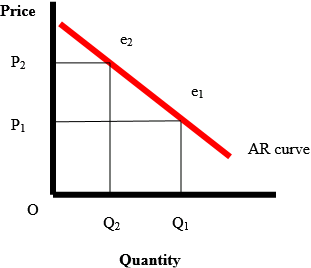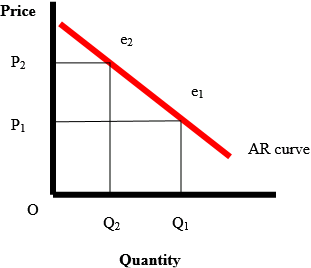Red line above is average revenue curve. Average revenue is nothing but price of the product. This curve is showing an inverse relation between average revenue and quantity. Suppose a firm was selling OQ ₁ units at OP 1 price. Now the firm has decided to charge higher price OQ ₂. At this price firm will be able to sell only OQ ₂ units. Thus a price searcher firm can set highest possible price. But it cannot decide the quantity to be sold. Both variables cannot be controlled at the same time. Maximum price of the profit maximizer: Maximum price of a profit maximizing firm will depend upon the profit maximizing output. At first, firm will fix up the profit maximizing quantity which it has to sell. Then from demand curve it has to accept the price which it will charge to sell the profit maximizing units. Profit Maximizing conditions: A price searcher like other firm has the basic objective of maximizing profit. In order to do so it has to fulfill understated conditions- 1. First condition of profit maximization requires that marginal revenue (MR) and marginal cost (MC) are equal. Marginal revenue is the extra money earned by selling last unit. On the other hand marginal cost will mean extra cost of manufacturing last unit. If marginal revenue is greater than marginal cost, then one unit extra sale will mean some addition in the total profit. So profit is not maximum when MR is greater than MC. Now consider opposite situation when MC is greater than MR. In this situation if extra unit is produced, then extra cost will exceed extra revenue. Thus profit will fall. Thus profit can be maximum only when they are equal. 2. Second condition requires that slope of marginal cost should be greater than the slope of marginal revenue. Note that MC curve is U shaped. But a firm cannot attain profit maximization in the falling portion of the curve since cost of last unit is decreasing. So by increasing production, firm will improve its profit. Thus profit maximization is possible only on the rising segment of MC curve where slope is positive. MR curve on the other hand has always a negative slope since AR curve is sloping downward. Thus profit maximization will take place when MC curve has a slope greater than the slope of MR curve. After fixing the profit maximizing quantity, Firm will look at the corresponding selling price from AR curve. This price will be the maximum price of a profit maximizing firm. Above idea can be explained with the help of understated diagram-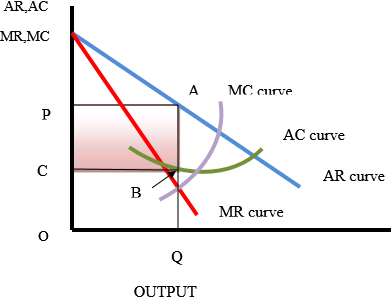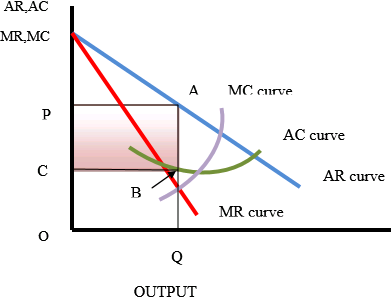Consider the diagram. It has a downward red colored demand or average revenue curve. Marginal revenue curve is below it. Marginal cost curve is passing through the minimum pint of average cost curve. Note that MC curve has intersected MR curve from below. Output is OQ at this cutting point. So OQ is profit maximizing point. Both conditions of profit maximization have been fulfilled at this output. Now extend the vertical line from Q. It has intersected AR curve at point A. Against this point A, price is OP. It is the maximum price which the profit maximizing firm can charge. The same line has intersected AC curve at point B. So average cost of manufacturing OQ units is BQ or OC. Here OC is less than OP. So economic profit per unit is length CP. Pink colored area is total economic profit at profit maximizing output.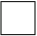To sum up maximum price of profit maximizing output quantity OQ is OP. Comparison of MC and profit maximizing price: At profit maximizing output OQ, marginal cost is found from the intersecting point 'e'. It will be always lower than the price OP. The reason is downward slope of the AR or demand curve. Since AR is falling with the increase in quantity, MR will be always less than price. As the profit maximization condition requires that MC should cut MR from below, it implies that MC should be always less than the profit maximizing price.
a. Price is affected by market condition: Concept of price searcher market: Price searchers market has the following features- 1. Many firm 2. Products manufactured are not same. They are close substitutes. Differentiation is created by advertisement, packaging, design change etc. Due to such differentiation even after price increase some customers remain loyal to the firm. They do not change the seller. 3. Entry of new firm and exit of existing firm is easy. So new firm will enter whenever there is scope of making high economic profit. Due to above properties, firm has some control over the price. It can decide the price at which product will be sold. Effect of demand curve on price and quantity: Setting of price does not mean that price is unaffected by market condition. Firm has to consider demand curve which is down ward sloping and highly elastic. If firm set the price then it cannot decide on the quantity to be sold. It will depend upon demand curve. If price set is high, then quantity will be low otherwise a portion of the quantity will remain unsold. Further demand curve is highly elastic. So market is very sensitive to price change. A little increase in price will reduce the quantity significantly. Thus market searcher has to set profit maximizing point only after considering the demand curve of the product in the market. It cannot fix both selling price and quantity at the same time. a. Economic profit in the long run: Price searchers market with low entry barriers can make economic profit, suffer abnormal loss or may earn only normal profit in the short run. But it is not possible in the long run. Only normal profit is possible in the long run. Concept of long run: In economy time can be grouped as- 1. Short run and 2. Long run. Short run is a time when everything cannot be changed according to requirements. At least one factor will remain constant. Production capacity or entrepreneurial skill cannot be changed in the short run. Long run, on the other hand, is a time when supply of all factors can be changed to adjust the production requirement. Concept of economic profit: It is the excess of total revenue over total cost. Total revenue is the total money value of quantity sold. Total cost includes payment to all factor owners for using the factors in production work. It includes normal profit which is considered as opportunity cost of entrepreneurs. If the normal profit is not realized, then entrepreneur will stop production and divert to some other best possible alternatives to realize that opportunity cost. If total revenue and total cost is equal, then firm will recover only normal profit. If total revenue exceeds total cost, then firm is earning economic profit. In opposite situation the firm is suffering abnormal loss. Suppose price searcher firm is making economic profit in the short run. It will attract many new firms to enter the industry. Since entry is easy, they will enter the business and will increase the supply of product. Also existing firm in the long run will increase the production capacity to manufacture more units so that profit can be increased. Thus total supply of the industry will rise. As the market has a downward sloping highly elastic demand curve, the price has to be cut. Otherwise produced quantity will not be sold in full. Due to cut in price, economic profit will gradually decrease. This process will continue until the entire economic profit is eliminated. The adjustment process as described can be explained with the help of understated diagram-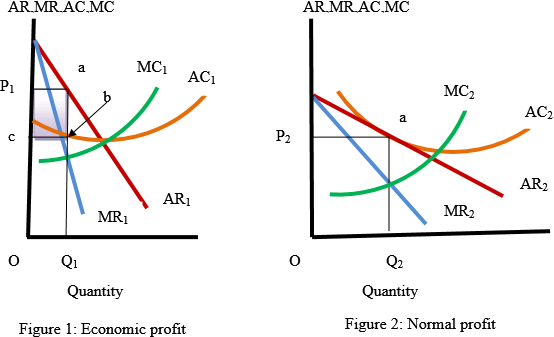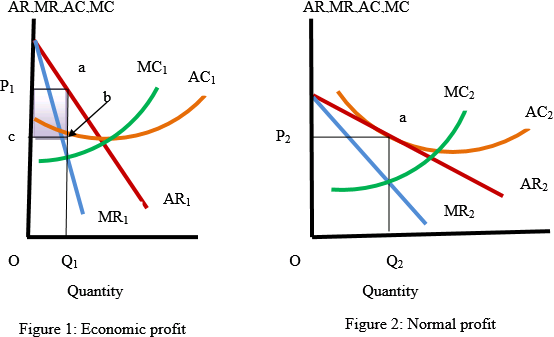In diagram 1, marginal cost curve MC 1 has intersected MR 1 curve at OQ ₁ quantity. It is profit maximizing quantity. Against this quantity, average cost is bQ ₁ and average revenue is aQ ₁. Therefore, ab is economic profit. Total economic profit is shown by the violet colored area P 1 abc. Now consider diagram 2. It is long run situation after entry of new firm and expansion of production capacity by the existing firm in the long run. Due to expansion in quantity supplied in the industry, AR 1 curve has shifted to AR 2. Also MR 1 curve has changed to MR 2 curve. Average cost curve has also changed to AC 2. Now MC 2 curve has intersected MR 2 curve at OQ ₂ units of production. Long run equilibrium price has fallen from OP 1 of diagram 1 to OP 2 in diagram 2. Both average revenue and average cost here is aQ ₂. Thus only normal profit is earned. Economic profit has been totally eliminated in the long run. c) Price searcher competitive firm has to take all possible effort to influence the customer. They have to add quality, style, variety, location etc. Also they can provide different types of services to attract them. Further they may spend lot of money on advertisement. All these efforts will convince customers that firm is capable of adding value to the product which customers like most. In this manner they will succeed in altering demand of their product. Customer of rival firm will shift in its favor. Turnover will go up. Firm will succeed in making economic profit.